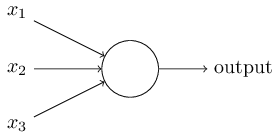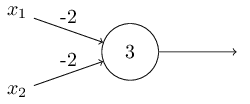# 开始

## Neural Networks & Deep Learning

Neural Networks, a beautiful biologically-inspired programming paradigm which enables a computer to learn from observational data.

Deep learning, a powerful set of techniques for learning in neural networks

# Perceptrons

So how do perceptrons work？简单来说，感知器得到几个二进制输入x1，x2，…，并输出单个二进制结果：$output = \begin{cases} 0, & \text {if \sum_i w_ix_i \leq 阈值} \\ 1, & \text{if \sum_i w_ix_i > 阈值} \end{cases}$

1. 你对薪水是否满意
2. 从你住的地方到公司的时间短吗
3. 公司的福利你满意吗$output = \begin{cases} 0, & \text {if W·X + b \leq 0} \\ 1, & \text{if W·X+ b > 0} \end{cases}$

$W = [w1, w2, w3]$

$X = [x1, x2, x3]^T$

b(bias) = -阈值